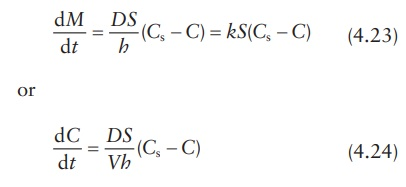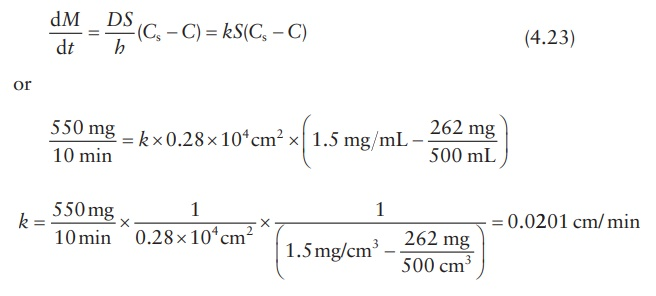# Dissolution

| Home | | Pharmaceutical Drugs and Dosage | | Pharmaceutical Industrial Management |

## Chapter: Pharmaceutical Drugs and Dosage: Biopharmaceutical considerations

For most drugs, the rate at which the solid drug dissolves in a solvent (dis-solution) is often the rate-limiting step in the drug’s bioavailability.

Dissolution

For most drugs, the rate at which the solid drug dissolves in a solvent (dis-solution) is often the rate-limiting step in the drug’s bioavailability.

## Noyes–Whitney equation

Noyes–Whitney equation correlates the dissolution rate of a drug with the particle surface area (S), the thickness of the unstirred solvent layer on the particle surface (h), diffusion coefficient of the drug (D), and the concentra-tion gradient, that is, the difference in the concentration of drug solution at the particle surface (Cs) and the bulk solution (C).where:

dM /dt is the mass rate of dissolution (mass of the drug dissolved per unit time, e.g., in mg/min)

D is the diffusion coefficient of solute in solution, in cm2/s

S is the surface area of the exposed solid, in cm2

k is the dissolution rate constant (k = D /h, in cm/s)

h is the thickness of the unstirred layer at the solid surface, in cm

Cs is the drug solubility at the particle surface, in g/mL

C is the drug concentration in bulk solution at time t, in g/mL

The quantity, dC/dt, represents the change in drug concentration in the bulk solution per unit time, or the dissolution rate, and V is the volume of solution (mL). Thus, C = M /V.

Under sink conditions, C << Cs. Therefore, the Noyes–Whitney equation can be simplified as:## Calculation example

Knowledge of dissolution rate constant, k, allows simulation of the rate of drug dissolution by using different quantities of drug substance, changes in the particle size and surface area of the drug, and dissolution condi-tions, such as volume. A simulation of these results can assist in dissolution method development by minimizing the number of experiments needed under different conditions. Dissolution rate constant can be calculated using dissolution data collected from a well-defined system.

For example, a preparation of drug particles weighing 550 mg and having a total surface area of 0.28 × 104 cm2 was allowed to dissolve in 500 mL of water at 37°C. Assuming that analysis of bulk dissolution sample showed that 262 g had dissolved after 10 min, if the saturation solubility of the drug in water is 1.5 mg/mL at 37°C, k can be calculated as follows:

According to the Noyes–Whitney equation,The dissolution rate constant is related to the diffusion constant of the drug through the solvent (D) and the diffusion layer thickness (h):

k = D/h        (4.25)

Therefore, if the diffusion layer’s thickness could be estimated, the diffu-sion coefficient of the drug can be calculated. Thus, if the diffusion layer’s thickness were 5 × 10–3 cm, the diffusion coefficient (D) would be given by:

0. 0201 cm/min = D / (5 × 10 3 cm)

or

D = 0. 0201 cm/min × 5 × 10 3 cm = 1.01×104 cm2 / min

## Factors influencing dissolution rate

The main biopharmaceutical and physiological factors that influence the dissolution rate of a drug can be summarized as follows:

1. Drug solubility: The greater the drug solubility, the greater the drug’s dissolution rate. This is evident in the Noyes–Whitney equation. The solubility and dissolution rates of acidic drugs are low in acidic gastric fluids, whereas the solubility and dissolution rates of basic drugs are high. Similarly, the solubility and dissolution rates of basic drugs are low in basic intestinal fluids, whereas those of acidic drugs is high.

2. Viscosity (of the dissolving medium): The greater the viscosity of the dissolving liquid, the lower the diffusion coefficient of the drug and hence the lower the dissolution rate. Viscosity of the dissolving bulk medium and/or the unstirred layer on the surface of the dissolving formulation can be affected by the presence of hydrophilic polymers in the formulation, which dissolve to form a viscous solution. In vivo, the viscosity may be affected by the food intake.

3. Diffusion layer’s thickness: The greater the diffusion layer’s thickness, the slower the dissolution rate. The thickness of the diffusion layer is influenced by the degree of agitation of the dissolving medium, both in vitro and in vivo. Hence, an increase in gastric and/or intestinal motility may increase the dissolution rate of poorly soluble drugs. For example, food and certain drugs can influence gastrointestinal (GI) motility.

4. Sink conditions: Removal rate of dissolved drugs by absorption through the GI mucosa and the GI fluid volume affect drug concen-tration in the GI tract.

5. pH (of the dissolving medium): The drug dissolution rate is deter-mined by the drug solubility in the diffusion layer surrounding each dissolving drug particle. The pH of the diffusion layer has a significant effect on the solubility of a weak electrolyte drug and its subsequent dissolution rate. The dissolution rate of a weakly acidic drug in GI fluid (pH 1–3) is relatively low because of its low solubility in the diffusion layer. If the pH in the diffusion layer could be increased, the solubility exhibited by the weak acidic drug in this layer (and hence the dissolution rate of the drug in GI fluids) could be increased. The potassium and sodium salt forms of the weakly acidic drug have a relatively high solubility at the elevated microenvironmental pH in the diffusion layer due to the strong counterion bases, KOH and NaOH, respectively. Thus, the dissolution of the drug particles takes place at a faster rate.

6. Particle size and surface area: An increase in the specific surface area (surface area per unit mass) of a drug in contact with GI fluids would increase its dissolution rate. Generally, the smaller a drug’s particle size, the greater its specific surface area and the higher the dissolution rate. However, particle size reduction may not always be helpful in increasing the dissolution rate of a drug and hence its oral bioavail-ability. For example:

·           Porosity of drug particles plays a significant role. Thus, smaller particles with lower porosity may have lower surface area com-pared with larger particles with greater porosity. The dissolution rate depends on the effective surface area, which includes the influence of particle porosity.

·           In some cases, particle size reduction may cause particle aggre-gation, thus reducing the effective surface area. To prevent the formation of aggregates, small drug particles are often dispersed in polyethylene glycol (PEG), polyvinylpyrrolidone (PVP), dextrose, or surfactants such as polysobrates. For example, micronized griseofulvin is dispersed in PEG 4000.

·           In addition, certain drugs such as penicillin G and erythromycin are unstable in gastric fluids and do not readily dissolve in them. For such drugs, particle size reduction may increase not only the rate of drug dissolution in gastric fluids but also the extent of drug degradation.

7. Crystalline structure: Amorphous (noncrystalline) forms of a drug may have faster dissolution rate compared with the crystalline forms. Some drugs exist in a number of crystal forms or polymorphs. These different forms may have significantly different drug solubility and dissolution rates.

a. Dissolution rate of a drug from a crystal form is a balance between the energy required to break the intermolecular bonds in the crys-tal and the energy released on the formation of the drug–solvent intermolecular bonds. Thus, stronger crystals may have lower intrinsic dissolution rate.

b. Intrinsic dissolution rate reflects the dissolution rate of a drug crystal or powder normalized for its surface area. It is expressed in terms of mass per unit time per unit surface area. Drug forms that have higher intrinsic dissolution rate are expected to have higher dissolution rates.

c. The greater strength of a crystalline polymorph, sometimes evi-dent by its high melting point and sometimes by the rank order, correlates with its lower intrinsic dissolution rate.

d. Similarly, amorphous solids, which lack a long-range order that defines crystalline structure, tend to have higher intrinsic dissolu-tion rates.

8. Temperature: An increase in temperature leads to greater solubil-ity of a solid, with positive heat of the solution. Heat of solution indicates release of heat on dissolving. Positive heat of solution is indicative of a greater strength of solute–solvent bonds formed (which release energy) compared with the solute–solute bonds bro-ken (which take energy). The solid will therefore dissolve at a more rapid rate if the system is heated. Therefore, in vitro dissolution studies are carried out at 37°C to simulate body temperature and in vivo dissolution condition.

9. Surfactants: Surface-active agents increase the dissolution rate by (a) lowering the interfacial tension, which lowers the contact angle of the solvent on the solid surface and increases wetting of the drug particle and penetration of the solvent inside the dosage form, and (b) increas-ing the saturation solubility of the drug in the dissolution medium. Surfactants such as sodium lauryl sulfate (SLS) and Triton X-100 are frequently used to achieve sink conditions and rapid dissolution during in vitro dissolution method development.

Contact Us, Privacy Policy, Terms and Compliant, DMCA Policy and Compliant

TH 2019 - 2022 pharmacy180.com; Developed by Therithal info.